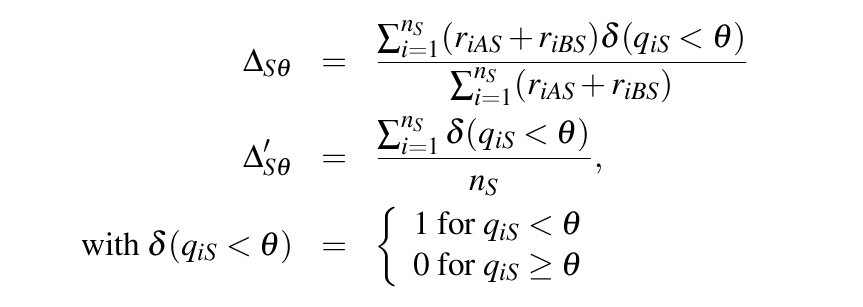discordance.delta {AmpliconDuo} R Documentation

Measure Of Discordance Between Two Amplicon Data Sets

Description

Calculates delta (Δ) and delta prime (Δ'), the fraction of amplicon frequencies and amplicons, respectively, with a false discovery rate below a certain threshold θ as a measure of discordance between two amplicon data sets A and B.

Usage

discordance.delta(x, names = NULL, theta = 0.05, corrected = TRUE,
printToTex = FALSE, directory = NULL, file.name = NULL)

Arguments

 x A list of amplicon duo data frames as returned by the ampliconduo function. names Optional. Vector or list of characters specifying the sample/amplicon pair names. By default names are taken from the element names of x. theta Optional. Numeric, threshold for the false discovery rate. Default value is 0.05. corrected Optional. Logical, indicates whether the p-value from Fisher's exact test (FALSE) or the adjusted p-value (TRUE), here called q, is used for calculation of Δ and Δ'. printToTex Optional. Logical, if TRUE writes a file with the resulting table in Tex format. Default is FALSE. directory Optional. If printToTex is TRUE, the output file will be saved in the specified directory. file.name Optional. If printToTex is TRUE, the output file will have the name file.name.

Details

Calculates Δ and Δ', the fraction of frequencies of amplicons and amplicons, respectively, with false discovery rate below a certain threshold θ as a measure of discordance between two amplicon data sets A and B with occurence r_{(iAS)} of amplicon i in amplicon set A of sample S (the ampliconduo data frame). Δ and Δ' are defined as follows:for number n_S of amplicons detected in sample/ampliconduo S. Δ_{Sθ} and Δ'_{Sθ} are located between 0 (no discordance, i.e. no statistically significant deviations between experimental branches) and 1 (complete discordance).

x is the return value of a ampliconduo call.

Value

Data frame with three columns. The first column contains the sample/amplicon pair names. Second and third column harbor the corresponding Δ and Δ' values, respectively.

Author(s)

Anja Lange & Daniel Hoffmann

ampliconduo, generates the expected data format for x

xtable, used to convert the returned data frame into a Latex table.

Examples

## load example ampliconduo data frame
data(amplicons)

## calculate the discordance between amplicon data sets of an ampliconduo data frame
dd.a <- discordance.delta(amplicons)
dd.b <- discordance.delta(amplicons, theta = 0.1)

[Package AmpliconDuo version 1.1.1 Index]